Next: Homogeneous Green Function Up: Klein-Gordon Equation Previous: Klein Paradox for Spin-0

# Coulomb Interaction

Consider the Coulomb interaction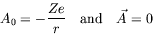(4.156)

and the substitution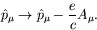(4.157)

The Klein-Gordon equation with a Coulomb potential is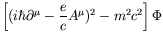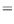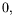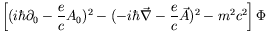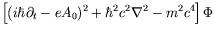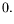(4.158)

For stationary states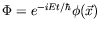we can write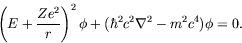(4.159)

For spherical coordinates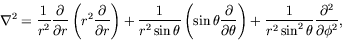(4.160)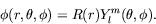(4.161)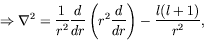(4.162)

where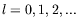. The Klein-Gordon equation in spherical coordinates is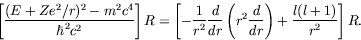(4.163)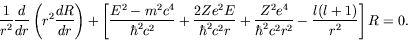(4.164)

Defining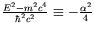we can write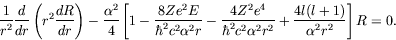(4.165)

Defining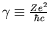we can write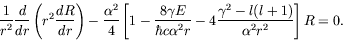(4.166)

Defining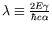we can write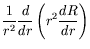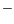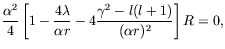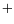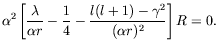(4.167)

Defining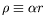and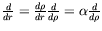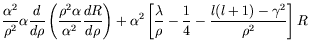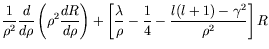(4.168)

This radial equation is the same as in the nonrelativistic case if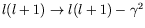. Solving for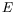we have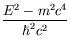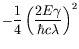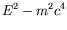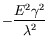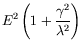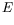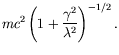(4.169)

The parameter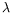is determined by the boundary condition on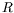when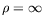.

The remainder of this problem is left as an exersize for the student.Next: Homogeneous Green Function Up: Klein-Gordon Equation Previous: Klein Paradox for Spin-0
Douglas M. Gingrich (gingrich@ ualberta.ca)
2004-03-18